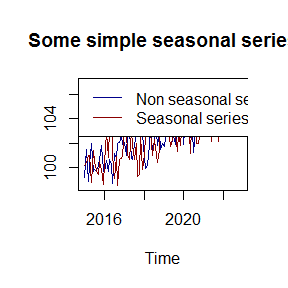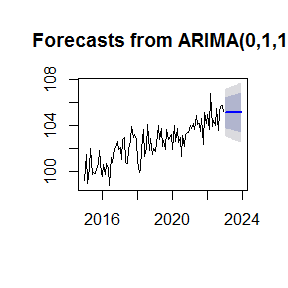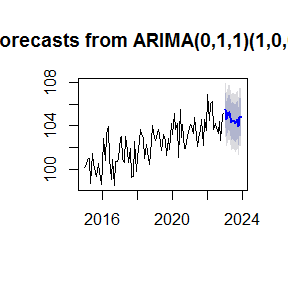# seastests - Seasonality tests

## Seasonality tests

Different authors have developed a multiplicity of test for seasonality of a given time series. In Ollech and Webel (forthcoming) and Webel and Ollech (forthcoming) we analysed many of these tests and showed a) which tests are the most reliable ones and b) how to combine a small set of tests, to reduce the misclassification rates of the single tests.

## To get started

We’ll simulated simple non-seasonal and a seasonal series.

set.seed(5)
x1 = 1:96/20 + ts(rnorm(96, 100, 1), start=c(2015,1), frequency=12)
x2 = 1:96/20 + ts(rnorm(96, 100, 1) + ts(sin((2*pi*rep(1:12, 8))/12), frequency=12), start=c(2015,1), frequency=12)

ts.plot(x1,x2, col=c("darkblue", "darkred"), main="Some simple seasonal series")
legend("topleft", c("Non seasonal series", "Seasonal series"), col=c("darkblue", "darkred"), lty=1)Then, we can test, whether these series are seasonal or not, using the WO-test, i.e. the overall seasonality test developed in Webel and Ollech (2019).

library(seastests)
print("Testing the non-seasonal series")
#>  "Testing the non-seasonal series"
summary(wo(x1))
#> Test used:  WO
#>
#> Test statistic:  0
#> P-value:  1 1 0.179945
#>
#> The WO - test does not identify  seasonality
print("")
#>  ""
print("Testing the seasonal series")
#>  "Testing the seasonal series"
summary(wo(x2))
#> Test used:  WO
#>
#> Test statistic:  1
#> P-value:  1 0.005145348 0.001151393
#>
#> The WO - test identifies seasonality

The WO-test gives out a TRUE if the series is seasonal and FALSE otherwise. The p-values indicate the p-values of the underlying test, i.e. the QS-test, the QS-R test and the KW-R-test.

If we are only interested in knowing, whether a series is seasonal or not, e.g. for further use in our analysis, the function isSeasonal() can be called.

print("Test using the non-seasonal series")
#>  "Test using the non-seasonal series"
isSeasonal(x1)
#>  FALSE
print("Test using the seasonal series")
#>  "Test using the seasonal series"
isSeasonal(x2)
#>  TRUE

This can then for example be used in the forecast packages auto.arima() function. For the non-seasonal series:

if (!require("forecast")) install.packages("forecast")
#> Loading required package: forecast
#> Warning: package 'forecast' was built under R version 3.5.1

mod1 <- auto.arima(x1, seasonal=isSeasonal(x1))
summary(mod1)
#> Series: x1
#> ARIMA(0,1,1)
#>
#> Coefficients:
#>           ma1
#>       -0.7597
#> s.e.   0.0656
#>
#> sigma^2 estimated as 1.085:  log likelihood=-138.6
#> AIC=281.2   AICc=281.33   BIC=286.31
#>
#> Training set error measures:
#>                     ME     RMSE       MAE       MPE     MAPE      MASE
#> Training set 0.2288794 1.030769 0.8455547 0.2146113 0.824723 0.6940177
#>                     ACF1
#> Training set -0.05882107
plot(forecast(mod1, h=12))and for the seasonal series:

mod2 <- auto.arima(x2, seasonal=isSeasonal(x2))
summary(mod2)
#> Series: x2
#> ARIMA(0,1,1)(1,0,0)
#>
#> Coefficients:
#>           ma1    sar1
#>       -0.8642  0.3531
#> s.e.   0.0502  0.1052
#>
#> sigma^2 estimated as 1.585:  log likelihood=-157.09
#> AIC=320.17   AICc=320.43   BIC=327.83
#>
#> Training set error measures:
#>                     ME     RMSE       MAE       MPE      MAPE     MASE
#> Training set 0.2134882 1.239136 0.9547015 0.1950671 0.9304067 0.777594
#>                    ACF1
#> Training set 0.03000515
plot(forecast(mod2, h=12))